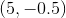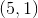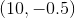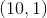# PSAT Math : Midpoint Formula

## Example Questions

← Previous 1

### Example Question #1 : How To Find The Midpoint Of A Line Segment

A line segment has endpoints (0,4) and (5,6). What are the coordinates of the midpoint?

(0,4)

(0,6)

(3,9)

(2.5,-5)

(2.5,5)

(2.5,5)

Explanation:

A line segment has endpoints (0,4) and (5,6). To find the midpoint, use the midpoint formula:

X: (x1+x2)/2 = (0+5)/2 = 2.5

Y: (y1+y2)/2 = (4+6)/2 = 5

The coordinates of the midpoint are (2.5,5).

### Example Question #2 : How To Find The Midpoint Of A Line Segment

Find the midpoint between (-3,7) and (5,-9)

(-1,-1)

(4,-1)

(4,-8)

(1,-1)

(1,-8)

(1,-1)

Explanation:

You can find the midpoint of each coordinate by averaging them.  In other words, add the two x coordinates together and divide by 2 and add the two y coordinates together and divide by 2.

x-midpoint = (-3 + 5)/2 = 2/2 = 1

y-midpoint = (7 + -9)/2 = -2/2 = -1

(1,-1)

### Example Question #3 : How To Find The Midpoint Of A Line Segment

Find the coordinates for the midpoint of the line segment that spans from (1, 1) to (11, 11).

(7, 7)

(5, 5)

(6, 6)

(6, 5)

(5, 6)

(6, 6)

Explanation:

The correct answer is (6, 6). The midpoint formula is ((x+ x2)/2),((y+ y2)/2) So 1 + 11 = 12, and 12/2 = 6 for both the x and y coordinates.

### Example Question #4 : How To Find The Midpoint Of A Line Segment

What is the midpoint between the points (–1, 2) and (3, –6)?

(–2,–1)

(1,2)

(–1,2)

(3,1)

(1,–2)

(1,–2)

Explanation:

midpoint = ((x1 + x2)/2, (y1 + y2)/2)

= ((–1 + 3)/2, (2 – 6)/2)

= (2/2, –4/2)

= (1,–2)

### Example Question #5 : How To Find The Midpoint Of A Line Segment

A line segment connects the points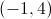and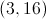. What is the midpoint of this segment?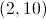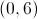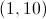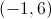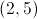Explanation:

To solve this problem you will need to use the midpoint formula: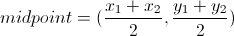Plug in the given values for the endpoints of the segment:and.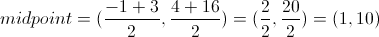### Example Question #1 : How To Find The Midpoint Of A Line Segment

What is the midpoint between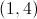and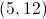?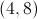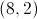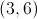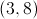Explanation:

The midpoint is the point halfway between the two endpoints, so sum up the coordinates and divide by 2: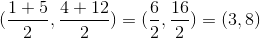### Example Question #7 : How To Find The Midpoint Of A Line Segment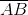has endpoints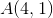and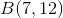What is the midpoint of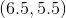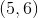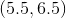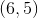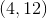Explanation:

The midpoint is simply the point halfway between the x-coordinates and halfway between the y-coordinates:

Sum the x-coordinates and divide by 2: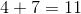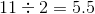Sum the y-coordinates and divide by 2: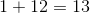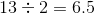Therefore the midpoint is (5.5, 6.5).

### Example Question #1 : How To Find The Endpoints Of A Line Segment

The midpoint of line segment AB is (2, -5).  If the coordinates of point A are (4, 4), what are the coordinates of B?

(6, 13)

(0, -13)

(6, 14)

(0, -14)

(3, -0.5)

(0, -14)

Explanation:

The fastest way to find the missing endpoint is to determine the distance from the known endpoint to the midpoint and then performing the same transformation on the midpoint.  In this case, the x-coordinate moves from 4 to 2, or down by 2, so the new x-coordinate must be 2-2 = 0.  The y-coordinate moves from 4 to -5, or down by 9, so the new y-coordinate must be -5-9 = -14.

An alternate solution would be to substitute (4,4) for (x1,y1) and (2,-5) for (x,y) into the midpoint formula:

x=(x1+x2)/2

y=(y1+y2)/2

Solving each equation for (x2,y2) yields the solution (0,-14).

### Example Question #2 : How To Find The Endpoints Of A Line Segment

Point A is (5, 7).  Point B is (x, y).  The midpoint of AB is (17, –4). What is the value of B?

(22, –9)

(12, –11)

(8.5, –2)

(29, –15)

(29, –15)

Explanation:

Point A is (5, 7). Point B is (x, y). The midpoint of AB is (17, –4). What is the value of B?

We need to use our generalized midpoint formula:

MP = ( (5 + x)/2, (7 + y)/2 )

Solve each separately:

(5 + x)/2 = 17 → 5 + x = 34 → x = 29

(7 + y)/2 = –4 → 7 + y = –8 → y = –15

Therefore, B is (29, –15).

### Example Question #3 : How To Find The Endpoints Of A Line Segment

Line segment AB has an endpoint, A, located at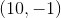, and a midpoint at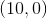. What are the coordinates for point B of segment AB?

The second endpoint cannot exist